Question

# A chemistry student weighs out 0.103g of sulfurous acid (H2SO3) , a diprotic acid, into a...

A chemistry student weighs out 0.103g of sulfurous acid (H2SO3) , a diprotic acid, into a 250.mL volumetric flask and dilutes to the mark with distilled water. He plans to titrate the acid with 0.0800M NaOH solution. Calculate the volume of NaOH solution the student will need to add to reach the final equivalence point. Be sure your answer has the correct number of significant digits.

Let us write the Balanced equation

H2SO3 + 2 NaOH ====> Na2SO3 + 2 H2O
Reaction type: double replacement.

Mass of sulfurous acid = 0.103 gm

Molar mass of sulfurous acid =  82.079 g/mol

Moles of sulfurous acid = 0.103 gm / 82.079 g/mol = 0.00125488 Moles

Moles of NaOH required = 0.00250977 Moles

Concentration of NaOH = 0.08 M

Volume of NaOH = 0.08 / 0.00250977 = 31.87 ml

Hence 31.87 ml of NaOH is required to neutralize 0.103 g of H2SO3.

#### Earn Coins

Coins can be redeemed for fabulous gifts.

Similar Homework Help Questions
• ### A chemistry student weighs out 0.115 g of sulfurous acid (H,SO,), a diprotic acid, into a...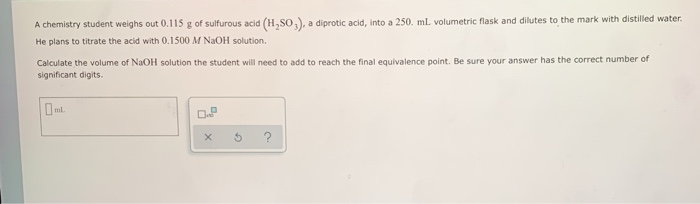A chemistry student weighs out 0.115 g of sulfurous acid (H,SO,), a diprotic acid, into a 250 mL volumetric flask and dilutes to the mark with distilled water. He plans to titrate the acid with 0.1500 M NaOH solution Calculate the volume of NaOH solution the student will need to add to reach the final equivalence point. Be sure your answer has the correct number of significant digits x 5 ?

• ### A chemistry student weighs out 0.140 g of sulfurous acid (H,SO2), a diprotic acid, into a...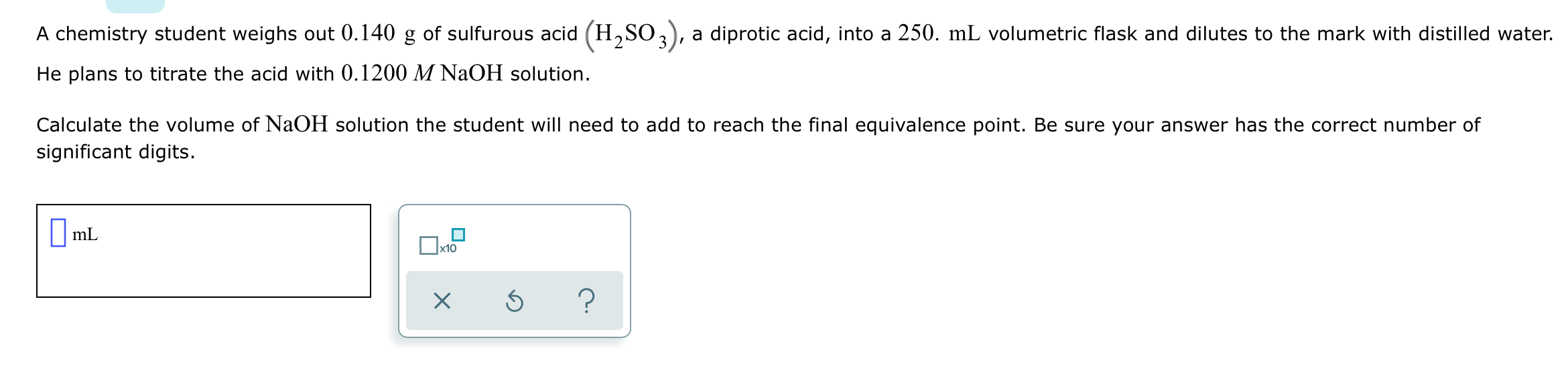A chemistry student weighs out 0.140 g of sulfurous acid (H,SO2), a diprotic acid, into a 250. mL volumetric flask and dilutes to the mark with distilled water. He plans to titrate the acid with 0.1200 M NaOH solution. Calculate the volume of NaOH solution the student will need to add to reach the final equivalence point. Be sure your answer has the correct number of significant digits. 1 ml x s ?

• ### A chemistry student weighs out 0.0768g of ascorbic acid H2C6H6O6, a diprotic acid, into a 250.mL...

A chemistry student weighs out 0.0768g of ascorbic acid H2C6H6O6, a diprotic acid, into a 250.mL volumetric flask and dilutes to the mark with distilled water. He plans to titrate the acid with 0.1500M NaOH solution. Calculate the volume of NaOH solution the student will need to add to reach the final equivalence point. Round your answer to 3 significant digits.

• ### A chemistry student weighs out 0.0873 g of formic acid (HCHO) into a 250. ml. volumetric...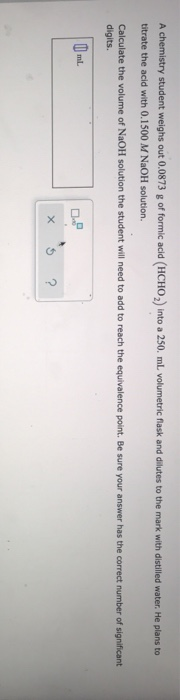A chemistry student weighs out 0.0873 g of formic acid (HCHO) into a 250. ml. volumetric flask and dilutes to the mark with distilled water. He plans to titrate the acid with 0.1500 M NaOH solution. Calculate the volume of NaOH solution the student will need to add to reach the equivalence point. Be sure your answer has the correct number of significant digits. ml X s ?

• ### A chemistry student weighs out 0.0609g of lactic acid HC3H5O3 into a 250.mL volumetric flask and...

A chemistry student weighs out 0.0609g of lactic acid HC3H5O3 into a 250.mL volumetric flask and dilutes to the mark with distilled water. He plans to titrate the acid with 0.1300M NaOH solution. Calculate the volume of NaOH solution the student will need to add to reach the equivalence point. Round your answer to 3 significant digits.

• ### A chemistry student weighs out 0.217g of hypobromous acid HBrO into a 250.mL volumetric flask and...

A chemistry student weighs out 0.217g of hypobromous acid HBrO into a 250.mL volumetric flask and dilutes to the mark with distilled water. He plans to titrate the acid with 0.0500M NaOH solution.Calculate the volume of NaOH  solution the student will need to add to reach the equivalence point. Round your answer to 3 significant digits.

• ### A chemistry student weighs out 0.117 g of citric acid (H.CHO), a triprotic acid, into a...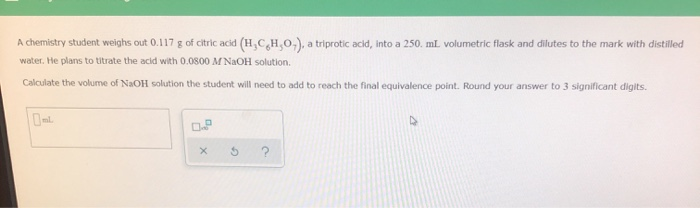A chemistry student weighs out 0.117 g of citric acid (H.CHO), a triprotic acid, into a 250 mL volumetric flask and dilutes to the mark with distilled water. He plans to titrate the acid with 0.0800 M NaOH solution Calculate the volume of NaOH solution the student will need to add to reach the final equivalence point. Round your answer to 3 significant digits.

• ### A chemistry student weighs out 0.0959 g of acrylic acid (HCH2CHCO2 into a 250. mL volumetric...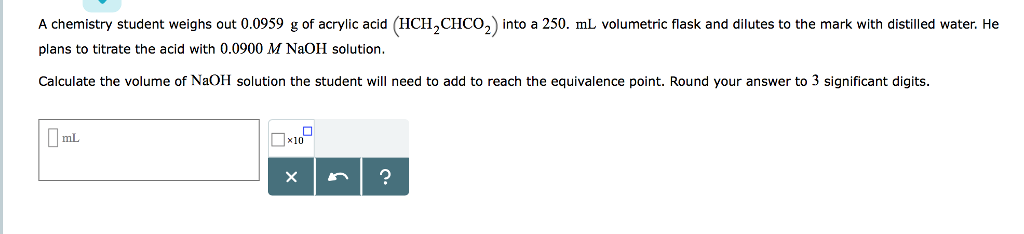A chemistry student weighs out 0.0959 g of acrylic acid (HCH2CHCO2 into a 250. mL volumetric flask and dilutes to the mark with distilled water. He plans to titrate the acid with 0.0900 M NaOH solution Calculate the volume of NaOH solution the student will need to add to reach the equivalence point. Round your answer to 3 significant digits. mL ×10

• ### A chemistry student weighs out 0.194g of chloroacetic acid HCH2ClCO2 into a 250.mL volumetric flask and...

A chemistry student weighs out 0.194g of chloroacetic acid HCH2ClCO2 into a 250.mL volumetric flask and dilutes to the mark with distilled water. He plans to titrate the acid with 0.0600M NaOH solution. Calculate the volume of NaOH solution the student will need to add to reach the equivalence point. Be sure your answer has the correct number of significant digits. Please put the correct decimal point in the final answer

• ### O SIMPLE REACTIONS Determining the volume of base needed to titrate a give... A chemistry student...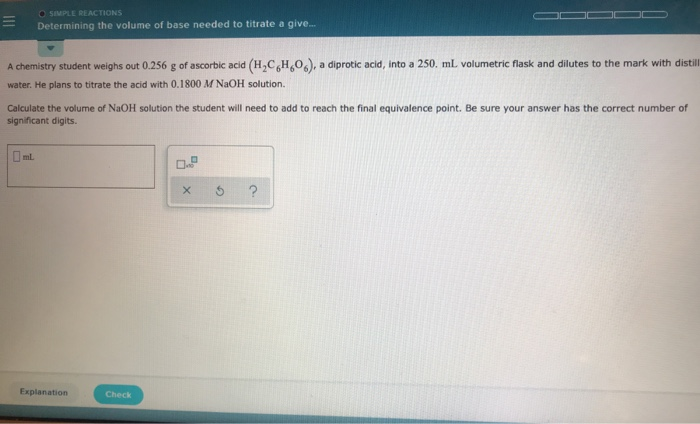O SIMPLE REACTIONS Determining the volume of base needed to titrate a give... A chemistry student weighs out 0.256 g of ascorbic acid (H2C6H06) water. He plans to titrate the acid with 0.1800 M NaOH solution. a diprotic acid, into a 250. mL volumetric flask and dilutes to the mark with distill Calculate the volume of NaOH solution the student will need to add to reach the final equivalence point. Be sure your answer has the correct number of significant...